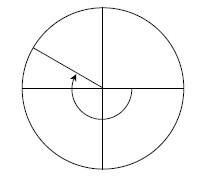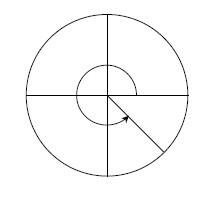### Home > PC > Chapter 3 > Lesson 3.2.3 > Problem3-94

3-94.

Below are two of the special angles that are used in a unit circle. Identify the radian measure for the angle shown. Remember counter-clockwise angles are positive and clockwise angles are negative.

1.1.Divide each circle into an equal number of parts. Remember that half of the circle is $π$ radians.